NCERT Solutions for Class 6 Maths Chapter 5 Understanding Elementary Shapes Ex 5.4 are part of NCERT Solutions for Class 6 Maths. Here we have given NCERT Solutions for Class 6 Maths Chapter 5 Understanding Elementary Shapes Ex 5.4.

 Board CBSE Textbook NCERT Class Class 6 Subject Maths Chapter Chapter 5 Chapter Name Understanding Elementary Shapes Exercise Ex 5.4 Number of Questions Solved 11 Category NCERT Solutions

## NCERT Solutions for Class 6 Maths Chapter 5 Understanding Elementary Shapes Ex 5.4

Question 1.
What is the measure of
(i) a right angle
(ii) a straight angle?
Solution :
(i) The measure of a right angle is 90°.
(ii) The measure of a straight angle is 180°.

Question 2.
Say True or False:
(a) The measure of an acute angle < 90°.
(b) The measure of an obtuse angle of < 90°.
(c) The measure of reflex angle > 180°.
(d) The measure of one complete revolution = 360°.
(e) If m∠A = 53° and m∠B = 35°, then m∠A > m∠B.
Solution :
(a) True
(b) False
(c) True
(d) True
(e) True.

Question 3.
Write down the measures of
(a) some acute angles.
(b) some obtuse angles. (give at least two examples of each).
Solution :
(a) 23°, 89°
(b) 91°, 179°.

Question 4.
Measure the angles given below, using the Protractor and write down the measure.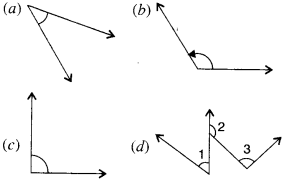Solution :
(a) 45°
(b) 125°
(e) 90°
(d) ∠l = 40°, ∠2 = 125° and ∠3 = 95°.

Question 5.
Which angle has a large measure? First, estimate and then measure.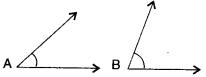Measure of angle A =
Measure of angle B =
Solution :
Measure of angle A = 40°
Measure of angle B = 65°
The angle B has a larger measure.

Question 6.
From these two angles which have larger measure? Estimate and then confirmed by the measuring them.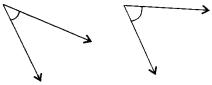Solution :
Measure of first angle = 45°
Measure of second angle = 60°
The second angle has larger measure.

Question 7.
Fill in the blanks with acute, obtuse, right or straight:
(a) An angle whose measure is less than that of a right angle is. ____________
(b) An angle whose measure is greater than that of a right angle is. ____________
(c) An angle whose measure is the sum of the measures of two right angles is. ____________
(d) When the sum of the measures of two angles is that of a right angle, then each one of them is. ____________
(e) When the sum of the measures of two angles is that of a straight angle and if one of them is acute than the other should be ____________.
Solution :
(a) An angle whose measure is less than that of a right angle is an acute angle.
(b) An angle whose measure is greater than that of a right angle is the obtuse angle (if the angle is less than 180°)
(c) An angle whose measure is the sum of the measures of two right angles is the straight angle.
(d) When the sum of the measures of two angles is that of a right angle, then each one of them is acute.
(e) When the sum of the measures of two angles is that of a straight angle and if one of them is acute than the other should be an obtuse angle.

Question 8.
Find the measure of the angle shown in each figure. (First, estimate with your eye, and then find the actual measurements with a protractor).
(a)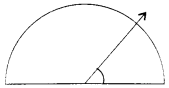(b)(c)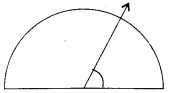(d)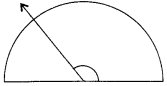Solution :
Estimation with eye
(a) 45°
(b) 125°
(c) 60°
(d) 135° actual measure with a protractor
Solution :
(a) 40°
(b) 130°
(c) 65°
(d) 135°

Question 9.
Find the angle measure between the hands of the clock in each figure :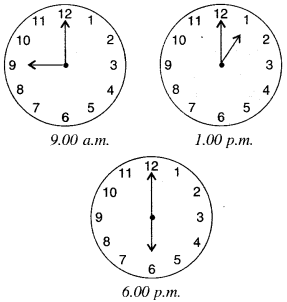Solution :

• 90°
• 30°
• 180°.

Question 10.
Investigate :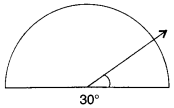In the given figure, the angle measures 30°. Look at the same figure through a magnifying glass. Does the angle become larger? Does the size of the angle change?
Solution :
No!; No!

Question 11.
Measure and classify each angle :Angle Measure Type ∠AOC ∠AOB ∠BOC ∠DOC ∠DOA ∠DOB

Solution :
Angle ∠AOB ∠AOC ∠BOC ∠DOC ∠DOA ∠DOB Measure 40° 120° 80° 105° 140° 180° Type acute obtuse acute obtuse straight.

We hope the NCERT Solutions for Class 6 Maths Chapter 5 Understanding Elementary Shapes Ex 5.4 help you. If you have any query regarding NCERT Solutions for Class 6 Maths Chapter 5 Understanding Elementary Shapes Ex 5.4, drop a comment below and we will get back to you at the earliest.